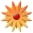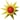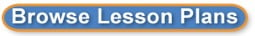# 7th grade- Adding Rational Numbers

Page Views: 3880

Email This Lesson Plan to Me
Email Address:
Subscribe to Newsletter?
Log in to rate this plan!
Overall Rating:(5.0 stars, 1 ratings)

 Keywords: Adding Rational numbers Subject(s): Grade 7 NETS-S Standard: Creativity and InnovationCommunication and CollaborationResearch and Information FluencyCritical Thinking, Problem Solving, and Decision MakingDigital CitizenshipTechnology Operations and ConceptsView Full Text of Standards School: Scoggins Middle School, Dallas, GA Planned By: angela bailey Original Author: angela bailey, Dallas
Grouping: Based on the results of the unit�s pre-test results
MCC7.NS.1 Apply and extend previous understandings of addition and subtraction to add and subtract rational numbers; represent addition and subtraction on a horizontal or vertical number line diagram.
MCC7. NS. 1a Describe situations in which opposite quantities combine to make 0
This week�s vocabulary- Absolute Value, Integer, Additive Inverse, Negative Numbers, Positive Numbers, Rational Numbers, and Zero Pairs
Monday-Aug 11
Objective 1: Students will apply and extend previous understandings of addition and subtraction to add and subtract rational numbers.
Objective 2: Students will describe situations in which opposite quantities combine to make 0.
Opening/Engage
Introduce the lesson with the following video:
https://learnzillion.com/lessons/673-adding-positive-and-negative-integers-on-a-number-line

Model
examples of absolute values and show how the absolute value sign is used.

Zero pairs represent additive inverses, a number and its opposite that equal zero. For example
+1 and -1 are opposites that equal zero when put together.
(a + - a = 0).
Review zero pair examples
Work-time/Explore/We Do-2 activities (stations)-Students will rotate to each station.
Station 1: Complete the following worksheet with your group members:
http://www.superteacherworksheets.com/integers/integers-number-line-addition1_TWRWR.pdf
(horizontal lines)

Station 2: Work-time/Explore/We Do- Students will complete Zip, Zilch, Zero (See the following link: http://illuminations.nctm.org ) (MCC7. NS. 1a Describe situations in which opposite quantities combine to make 0)
Reteach: I will create an additional small group to work with students that need additional assistance.
Closing/Explain- Explain in your own words what you believe zero pairs are. Use examples and describe one real life situation where zero pairs are used. Then explain why the absolute value of a number is always positive. Create one real world problems to demonstrate adding positive and negative numbers.
Tuesday Aug. 12th

MCC7.NS.1b Understand p + q as the number located a distance q from p, in the positive or negative direction depending on whether q is positive or negative. Show that a number, and its opposite, have a sum of 0 (are additive inverses). Interpret sums of rational numbers by describing real-world contexts.
Pre-assessment: Add the following integers using a number line. A. -5+10, B. 10+ (-18)
Objective: Students will add integers using a number line.
Opening/Engage- Introduce addition of integers using vertical and horizontal number lines.

Ex. 4 + (-3).
Start at 0-Move in the positive direction of the first number (4 spaces) and put a point on the number line at that spot. Move in the negative direction of the second number �3. Put another point at that spot on the number line. Where did you end up? +1. When you are counting, always remember to include the 0. (When you are counting, always remember to include the 0) Review the six problems listed below:

1:-2 + 6 = 4

#2:-2 + 8 =

#3:-6 + 9 =

#4:-4 + 6 =

#5:-9 + 3 =

#6:-5 + 2 =

Work-time/Explore/We Do
http://www.superteacherworksheets.com/integers/integers-number-line-addition1_TWRWR.pdf
(horizontal lines)
Using http://illuminations.nctm.org complete the following activity: Using an Elevator to Evaluate Signed Number Expressions: Flipping for Integers (Vertical lines)
Reteach: Small group-I will review the number line (negative and positive). I will review how to add integer
Closing/Explain/You Do (Post-Assessment)-Students will create their own problems involving addition of integers.

Wednesday Aug 13th
Pre-Assessment- Students will solve 3 adding negative and positive numbers using the chip/counter method. (MUST USE THE CHIP/COUNTER METHOD)
(Put answer on an index card and put in the basket next to the door)
MCC7.NS.1b Understand p + q as the number located a distance q from p, in the positive or negative direction depending on whether q is positive or negative. Show that a number and its opposite have a sum of 0 (are additive inverses)-Interpret sums of rational numbers by describing real-world contexts.
Objective: Students will solve problems involving addition of integers using the Chip/Counter Method.
Opening/Engage/I Do
Introduce adding integers using chips/counter method. Show the video listed below:
https://learnzillion.com/lessons/1023-add-integers-using-color-counters

Work-time/Explore/We
Do

USE COUNTERS TO FIND EACH SUM. DRAW A PICTURE OF YOUR ANSWER.
1. 5 +6
2. -3 + (-5)
3. -6 + 5
4. 3 + (-6)
5. -4 + 10

Closing/Explain/You Do (Post-Assessment)

It Looks Like This-An actual object or model that directly relates to the lesson is shown and students explain how it connects to the day�s concept.
Students will explain how the teacher got the answer to a problem displayed on the board. (Ex: -3+5=2) Students will need to explain using all three of the follow methods:
a. Number lines
b. Counters
c. Summary/Explanation in words

Thursday Aug 14th
Pre-Assessment: (use numbers less than 20) Using as Real World Problem, explain how to add integers using the Counter Method, vertical line, and horizontal line. (Put answer on an index card and put in the basket next to the door)
Objective: Review Zero Pairs, Adding Integers using horizontal lines, vertical lines, and counters.

Opening/Explore/I Do-

Students that understand the concepts listed in the objective will play the Integer Card Game-(use games to reinforce concepts)

Students that need additional assistance will work in small groups with the teacher.

Friday Aug. 15th Quiz

 Materials: Whiteboards